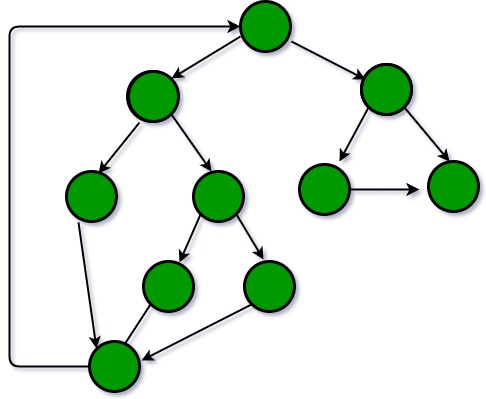Related Articles

# GATE | GATE-CS-2015 (Set 1) | Question 65

• Difficulty Level : Easy
• Last Updated : 14 Feb, 2018

Consider the following C program segment.

 `while` `(first <= last)``{``   ``if` `(array [middle] < search)``      ``first = middle +1;``   ``else` `if` `(array [middle] == search)``      ``found = True;``   ``else` `last = middle – 1;``   ``middle = (first + last)/2;``}``if` `(first < last) not Present = True;`

The cyclomatic complexity of the program segment is __________.

(A) 3
(B) 4
(C) 5
(D) 6

Explanation: the cyclomatic complexity of a structured program[a] is defined with reference to the control flow graph of the program, a directed graph containing the basic blocks of the program, with an edge between two basic blocks if control may pass from the first to the second. The complexity M is then defined as

```
M = E − N + 2P,
where
E = the number of edges of the graph.
N = the number of nodes of the graph.
P = the number of connected components.  ```

For a single program (or subroutine or method), P is always equal to 1. So a simpler formula for a single subroutine is

`    M = E − N + 2 `

For the given program, the control flow graph is:``` E = 13, N = 10.

Therefore, E - N + 2 = 5. ```

Attention reader! Don’t stop learning now.  Practice GATE exam well before the actual exam with the subject-wise and overall quizzes available in GATE Test Series Course.

Learn all GATE CS concepts with Free Live Classes on our youtube channel.

My Personal Notes arrow_drop_up